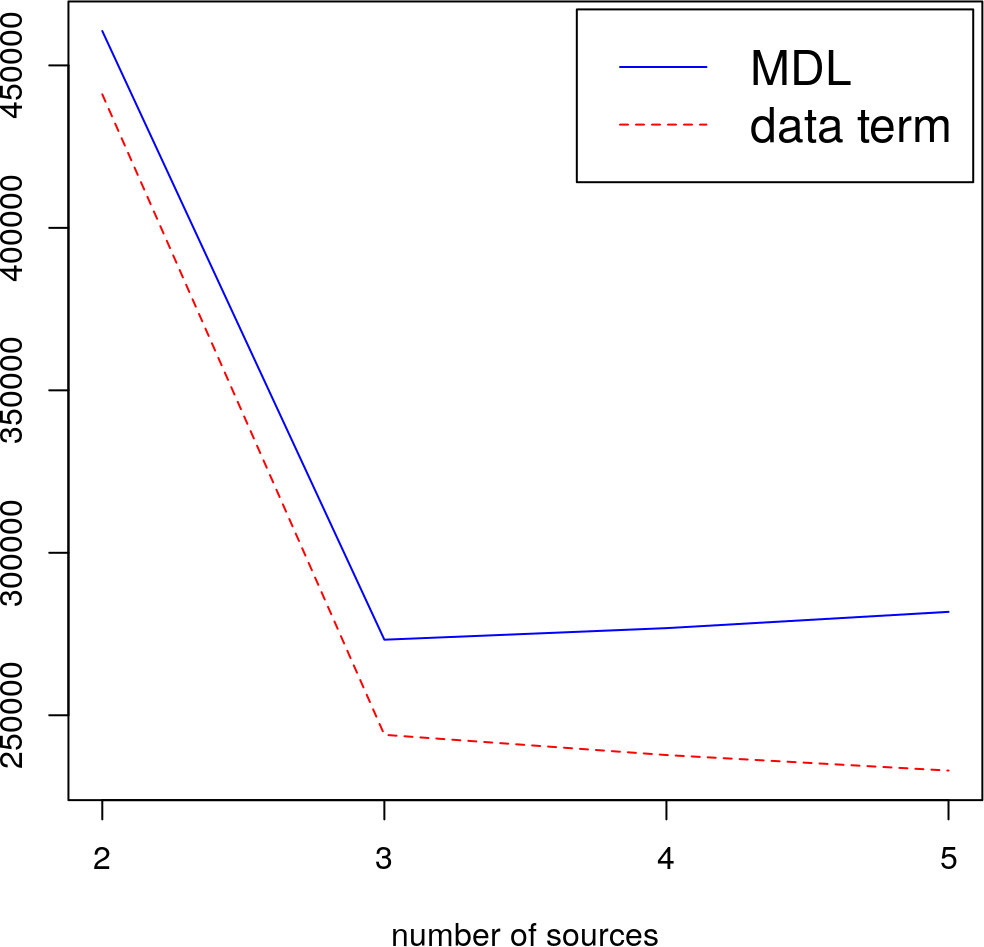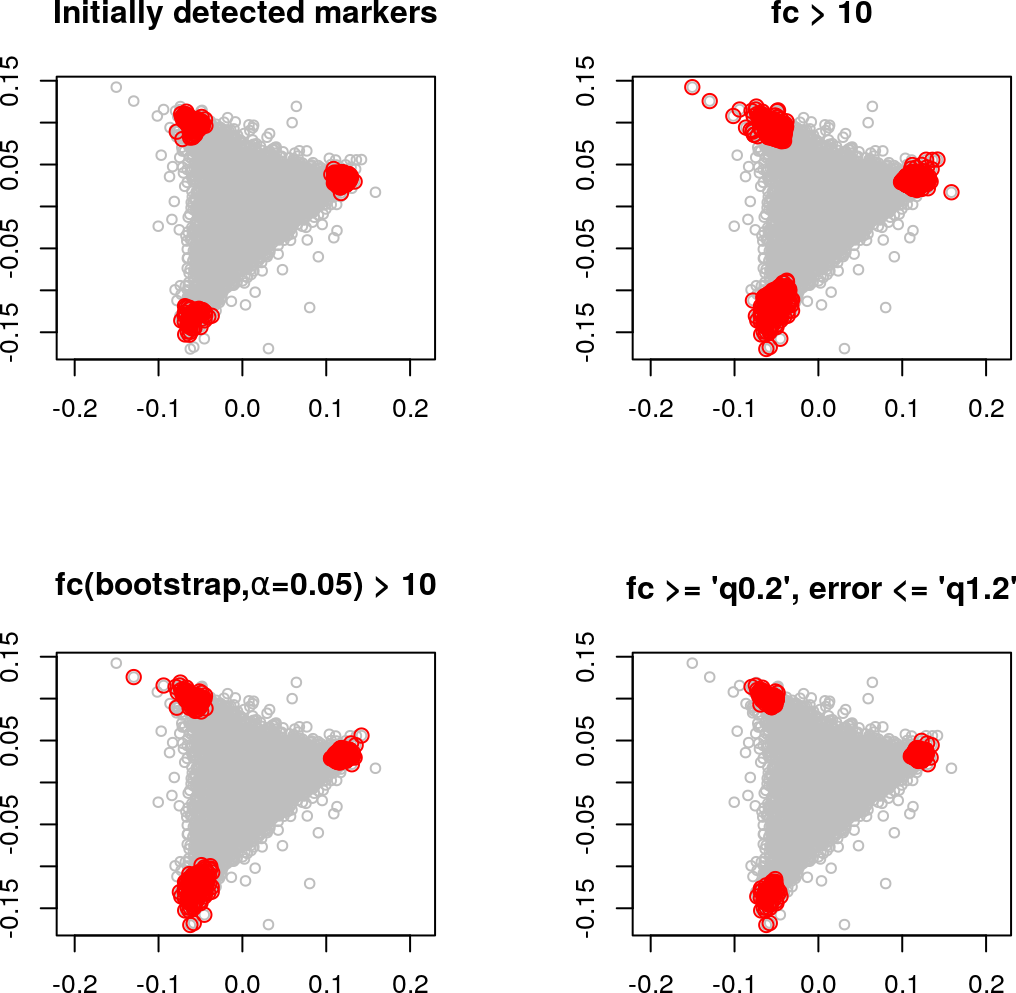# 1 Introduction

Convex Analysis of Mixtures (CAM) is a fully unsupervised computational method to analyze tissue samples composed of unknown numbers and varying proportions of distinct subpopulations (Wang et al. 2016). CAM assumes that the measured expression level is the weighted sum of each subpopulation’s expression, where the contribution from a single subpopulation is proportional to the abundance and specific expression of that subpopulation. This linear mixing model can be formulated as $$\mathbf{X'=AS'}$$. CAM can identify molecular markers directly from the original mixed expression matrix, $$\mathbf{X}$$, and further estimate the constituent proportion matrix, $$\mathbf{A}$$, as well as subpopulation-specific expression profile matrix, $$\mathbf{S}$$.

CAMTHC is an R package developed for tissue heterogeneity characterization by CAM algorithm. It provides basic functions to perform unsupervised deconvolution on mixture expression profiles by CAM and some auxiliary functions to help understand the subpopulation-specific results. CAMTHC also implements functions to perform supervised deconvolution based on prior knowledge of molecular markers, S matrix or A matrix. Semi-supervised deconvolution can also be achieved by combining molecular markers from CAM and from prior knowledge to analyze mixture expressions.

# 2 Quick Start

The function CAM() includes all necessary steps to decompose a matrix of mixture expression profiles. There are some optional steps upstream of CAM() that downsample the matrix for reducing running time. Each step in CAM() can also be performed separately if you prefer a more flexible workflow. More details will be introduced in sections below.

Starting your analysis by CAM(), you need to specify the range of possible subpopulation numbers and the percentage of low/high-expressed molecules to be removed. Typically, 30% ~ 50% low-expressed genes can be removed from gene expression data. Much less low-expressed proteins are removed, e.g. 0% ~ 10%, due to a limited number of proteins in proteomics data. The removal of high-expressed molecules has much less impact on results, usually set to be 0% ~ 10%.

rCAM <- CAM(data, K = 2:5, thres.low = 0.30, thres.high = 0.95)

# 3 Unsupervised Deconvolution

## 3.1 Datatypes and Input Format

Theoretically, CAMTHC accepts any molecular expression data types as long as the expressions follow the linear mixing model. We have validated the feasibility of CAM in gene expression data (microarray, RNAseq), proteomics data and DNA methylation data. Requirements for the input expression data:

• be in non-log linear space with non-negative numerical values (i.e.>=0).
• be already processed by normalization and batch effect removal.
• no missing values; the molecules containing missing values should be removed prior to CAM.
• no all-zero expressed molecules; otherwise, all-zero expressed molecules will be removed internally.

The input expression data should be stored in a matrix. Data frame, SummarizedExperiment or ExpressionSet object is also accepted and will be internally coerced into a matrix format before analysis. Each column in the matrix should be a tissue sample. Each row should be a probe/gene/protein/etc. Row names should be provided so that CAM can return the names of detected markers. Otherwise, rows will be automatically named by 1,2,3,…

## 3.2 CAM Workflow

We use a data set downsampled from GSE19830 as an example to show CAM workflow. This data set provides gene expression profiles of pure tissues (brain, liver, lung) and their biological mixtures with different proportions.

library(CAMTHC)
#> Warning: Package 'CAMTHC' is deprecated and will be removed from Bioconductor
#>   version 3.12 . Package renamed to debCAM

data(ratMix3)
#ratMix3$X: X matrix containing mixture expression profiles to be analyzed #ratMix3$A: ground truth A matrix containing proportions
#ratMix3$S: ground truth S matrix containing subpopulation-specific expression profiles data <- ratMix3$X
#10000 genes * 21 tissues
#meet the input data requirements

### 3.2.1 Analysis by CAM

Unsupervised deconvolution can be achieved by the function CAM() with a simple setting as Section 2 introduced. Other critical parameters are dim.rdc (reduced data dimension) and cluster.num (number of clusters). Increasing them will bring more time complexity. We can also specify cores for parallel computing configured by BiocParallel. cores = 0 will disable parallel computing. No cores argument will invoke one core for each element in K. Setting the seed of the random number generator prior to CAM can generate reproducible results.

set.seed(111) # set seed for internal clustering to generate reproducible results
rCAM <- CAM(data, K = 2:5, thres.low = 0.30, thres.high = 0.95)
#CAM return three sub results:
#rCAM@PrepResult contains details corresponding to data preprocessing.
#rCAM@MGResult contains details corresponding to marker gene clusters detection.
#rCAM@ASestResult contains details corresponding to A and S matrix estimation.

### 3.2.2 Model Selection by MDL Curves

We used MDL, a widely-adopted and consistent information theoretic criterion, to guide model selection. The underlying subpopulation number can be decided by minimizing the total description code length:

plot(MDL(rCAM), data.term = TRUE)### 3.2.3 Estimated A and S matrix

The A and S matrix estimated by CAM with a fixed subpopulation number, e.g. K = 3, can be obtained by

Aest <- Amat(rCAM, 3)
Sest <- Smat(rCAM, 3)

### 3.2.4 Detected Marker Genes

The marker genes detected by CAM and used for A matrix estimation can be obtained by

MGlist <- MGsforA(rCAM, K = 3) #for three subpopulations

Data preprocessing has filtered many genes, among which there are also some biologically-meaningful marker genes. So we need to check each gene again to find all the possible markers. Two statistics based on the subpopulation-specific expressions are exploited to identify marker genes with certain thresholds. The first is OVE-FC (one versus everyone - fold change) (Yu et al. 2010). The second is the lower confidence bound of bootstrapped OVE-FC at $$\alpha$$ level.

MGstat <- MGstatistic(data, Aest, boot.alpha = 0.05, nboot = 1000)
MGlist.FC <- lapply(seq_len(3), function(x)
rownames(MGstat)[MGstat$idx == x & MGstat$OVE.FC > 10])
MGlist.FCboot <- lapply(seq_len(3), function(x)
rownames(MGstat)[MGstat$idx == x & MGstat$OVE.FC.alpha > 10])

The thresholds above are set arbitrary and will impact the number of resulted markers significantly. Each subpopulation can also have different threshold. To make threshold setting more easier, we can also set thresholds to be quantile of fold changes and margin-of-errors of one subpopulation’s input markers. Margin-of-error is the distance between one gene and the simplex defined by column vectors of A matrix (Wang et al. 2016). Margin-of-error threshold can be relaxed to a value larger than the maximum.

MGlist.re <- reselectMG(data, MGlist, fc.thres='q0.2', err.thres='q1.2')
#q0.2: 0.2-quantile
#q1.2: 1-quantile (maximum) times 1.2

### 3.2.5 (Optional) Re-estimation

It is optional to re-estimate A and S matrix based on the new marker list and/or apply Alternating Least Square (ALS) method to further reduce mean squared error. Note that allowing too many iterations of ALS may bring the risk of a significant deviation from initial values. The constraint for methylation data, $$\mathbf{S\in[0,1]}$$, will be imposed during re-estimation.

rre <- redoASest(data, MGlist.re, maxIter = 2, methy = FALSE)
#rre$Aest: re-estimated A matrix #rre$Sest: re-estimated S matrix

### 3.2.6 Simplex Plot - 2D

Fundamental to the success of CAM is that the scatter simplex of mixed expressions is a rotated and compressed version of the scatter simplex of pure expressions, where the marker genes are located at each vertex. simplexplot() function can show the scatter simplex and detected marker genes in a 2D plot. The vertices in the high-dimensional simplex will still locate at extreme points of the low-dimensional simplex.

layout(matrix(c(1,2,3,4), 2, 2, byrow = TRUE))
simplexplot(data, Aest, MGlist, main=c('Initially detected markers'))
simplexplot(data, Aest, MGlist.FC, main=c('fc > 10'))
simplexplot(data, Aest, MGlist.FCboot,
main=c(expression(bold(paste('fc(bootstrap,',alpha,'=0.05) > 10')))))
simplexplot(data, Aest, MGlist.re, main=c("fc >= 'q0.2', error <= 'q1.2'"))The colors and the vertex order displayed in 2D plot can be changed as

simplexplot(data, Aest, MGlist.FCboot,
data.extra=rbind(t(ratMix3\$A),t(Aest)),
corner.order = c(2,1,3), col = "blue",
mg.col = c("red","orange","green"),
ex.col = "black", ex.pch = c(19,19,19,17,17,17))
legend("bottomright", cex=1.2, inset=.01, c("Ground Truth","CAM Estimation"),
pch=c(19,17), col="black")